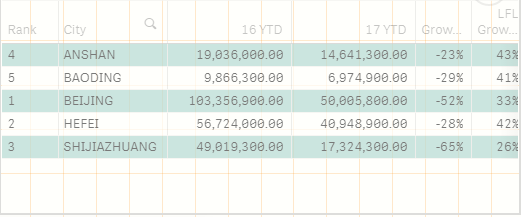Qlik Sense App Development

New Contributor

Why is the result of my expression 0?

Hi, everyone, I'm a beginner in learning qlik, and on sense desktop I had a problem doing an exercise with an example: when I created a table and wrote an expression in it, my results were different from those in the example, but our scripting language, expressions, and data They are all the same.and this is my  expression :sum({<TxDate={'2016*'}>} SalesAmount)

1 Solution

Accepted Solutions
Highlighted
Valued Contributor

Re: Why is the result of my expression 0?

Try This

sum({<TxDate={">=\$(=makedate(2016,01,01))<=\$(=makedate(2016,12,31))"}>} SalesAmount)

5 Replies
Valued Contributor

Re: Why is the result of my expression 0?

Because TxDate is date

and qlik consider date as numeric field not string

Highlighted
Valued Contributor

Re: Why is the result of my expression 0?

Try This

sum({<TxDate={">=\$(=makedate(2016,01,01))<=\$(=makedate(2016,12,31))"}>} SalesAmount)

New Contributor

Re: Why is the result of my expression 0?MVP

Re: Why is the result of my expression 0?

Please mark the answer as correct to close the threadValued Contributor

Welcome